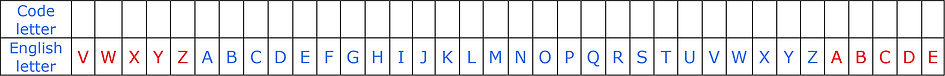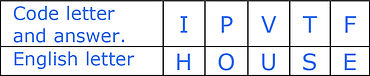# 11+ Verbal Reasoning

## ​

There are a wide variety of possible codes that can be used in this question type. Some of these codes can be very complicated while others are much simpler and easier to work out.

In this question type you may be asked to change code words into English or an English word into a code word.

Look at this first example and then we can work our way through the question until we get the answer: -

In a certain code the word HOME is written as IPNF.

What is HOUSE in code?

It will be useful to have the alphabet printed in a form something like this.Notice that we have added the next five letters to the start and end of the alphabet, we have added these letters in RED for ease of use.

Sometimes codes can go past the start or end of the alphabet. This may be important and is something that you should be aware of from the start of this exercise.Using the above table we have put the code letters above the correct letters for the word HOME.

The letters of the word HOME are coloured
GREEN.

When we look carefully at our table we can see that the code uses the next letter in the alphabet –

H becomes I

O becomes P
and so on.We have now coloured the word HOUSE in GREEN to help us work out the code which we now know uses the next letter in the alphabet.The simple table above helps us to create our answer to:-

What is HOUSE in code?

Look at this second example and then we can work our way through the question until we get the answer: -

In a certain code the word CHILD is written as EJKNF.

What is the English word for the code IKTN?Using the above table we have put the code letters above the correct letters for the word CHILD. The letters of the word CHILD are coloured GREEN.

When we look carefully at our table we can see that the code uses the letter that is two letters forward in the alphabet –

C becomes E

H becomes J

and so on.This time we have to change an English word into a code word.

We have worked out the code which we now know uses the letter that is two letters forward in the alphabet.

So moving from code to an English word we must go two letters BACKWARDS in the alphabet –

I becomes G

K becomes I

T becomes R

N becomes L

In the above table the letters of the English word has been coloured GREEN.The simple table above helps us to create our answer to:-

What is the English word for the code IKTN?

Look at this third example and then we can work our way through the question until we get the answer: -

In a certain code the word SCARE is written as ABCDX.

What is the English word for the code DCBX?

In this example all the letters of the word SCARE are used in the code ABCDX.

We can enter the CODE and English word into a simple table and then answer the question.We have coloured the coded word DCBX in GREEN.

We can see that

D becomes R

C becomes A

and so on

giving us the word RACE.

## ​

• Make sure that you are going from code to English or English to code as required by the question.

• Work systemically through the question using some sort of grid or table that makes sense to you.

• Remember that the code may go past the end or start of the alphabet.

• There is a huge variety of codes that are possible in this question type.

NB When you are completing a Verbal Reasoning test in Multiple-Choice format the correct answer will always be one of the choices that you are given on your answer sheet. If your answer does not match one of the answers that you are given then your answer is wrong.